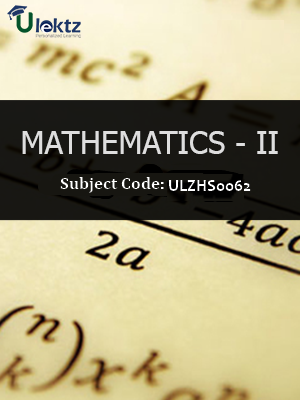•My WalletMy Order
•My Profile
•My Connections
•My Books
•My Videos
•My Tests
•My Calender
•My Messages
•My Shopping Cart
•My Orders
•Account Settings
•Help

# Book Details# Mathematics-II

 Course Code : ULZHS0062 Author : uLektz University : General for All University Regulation : 2013 Categories : Electronics & Communication Format :ePUB3 (DRM Protected) Type : eBook

FREE

Description :Mathematics-II of ULZHS0062 covers the latest syllabus prescribed by General for All University for regulation 2013. Author: uLektz, Published by uLektz Learning Solutions Private Limited.

Note : No printed book. Only ebook. Access eBook using uLektz apps for Android, iOS and Windows Desktop PC.

##### Topics
###### UNIT I ORDINARY DIFFERENTIAL EQUATION AND ITS APPLICATIONS

1.1 Exact differential equation of first order, Equations reducible to exact differential equation

1.2 Differential equation of second and higher order - Complete solutions of linear differential equations(Complementary Function + Particular Integral)

1.3 Method of variation of parameter to find Particular Integral

1.4 Cauchy’s and Legendre’s linear Equation

1.5 Simultaneous linear equations with constant co-efficient

1.6 Application of linear differential equations to Electric circuits (LC,LCR circuit), Newton’s law of cooling, Heat flow, Orthogonal trajectory

###### UNIT II LAPLACE TRANSFORMS AND ITS APPLICATIONS

2.1 Laplace-transforms of elementary functions, Elementary properties of Laplace-transforms, Existence conditions

2.2 Transforms of derivatives, Transforms of integral, Multiplications by tn, Division by t

2.3 Evaluation of integrals by Laplace transforms, Second shifting Theorem

2.4 Inverse transforms

2.5 Convolution theorem

2.6 Applications to linear differential equations to solve boundary value problems with constants coefficients and simultaneous linear differential equations with constant coefficients

###### UNIT III PARTIAL DIFFERENTIAL EQUATION AND ITS APPLICATION

3.1 Formation of partial differential equations, Lagrange’s linear partial differential equations

3.2 First order non-linear partial differential equations, Charpit’s method

3.3 Homogeneous Partial differential equation of second and higher order

3.4 Method of Separation of Variables and its applications to wave equation and one dimensional Heat equation

###### UNIT IV INFINITE SERIES

4.1 Convergence and divergence of Infinite series, Comparison Test, D’Alembert’s Ratio Test

4.2 Gauss Test, Integral Test, Raabe’s Test

4.3 Logarithmic Test, Cauchy’s Root Test

4.4 Alternating series, Conditional Convergence & Absolute Convergence